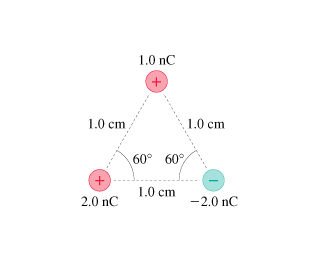# Problem: What is the force F on the 1.0 nC charge in (Figure 1)?A) Determine the magnitude of the force F.B) Determine the direction of the force F.

###### FREE Expert Solution

The electric force from Coulomb's law:

$\overline{){\mathbf{F}}{\mathbf{=}}\frac{\mathbf{k}{\mathbf{q}}_{\mathbf{1}}{\mathbf{q}}_{\mathbf{2}}}{{\mathbf{r}}^{\mathbf{2}}}}$, where k is Coulomb's constant and r is the distance between two charges q1 and q2.

The net electric force is expressed as:

$\overline{){{\mathbf{F}}}_{\mathbf{n}\mathbf{e}\mathbf{t}}{\mathbf{=}}\sqrt{{{\mathbf{F}}_{\mathbf{x}}}^{\mathbf{2}}\mathbf{+}{{\mathbf{F}}_{\mathbf{y}}}^{\mathbf{2}}}}$, where Fx and Fy are the x and y components of electric force.

Direction:

$\overline{){\mathbf{\theta }}{\mathbf{=}}{\mathbf{t}}{\mathbf{a}}{{\mathbf{n}}}^{\mathbf{-}\mathbf{1}}{\mathbf{\left(}}\frac{{\mathbf{F}}_{\mathbf{y}}}{{\mathbf{F}}_{\mathbf{x}}}{\mathbf{\right)}}}$

A.

In this problem, let's consider the direction to the right to be positive x-axis and upwards to be positive y-axis.

The force F1 due to charge q1 on charge q2 is given by:

97% (25 ratings)###### Problem DetailsWhat is the force F on the 1.0 nC charge in (Figure 1)?

A) Determine the magnitude of the force F.

B) Determine the direction of the force F.